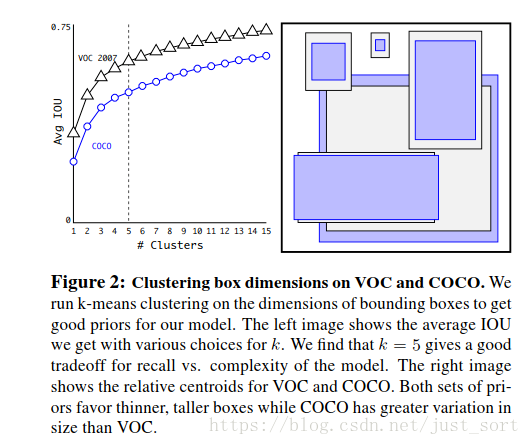# 原理¶

## Anchor如何获得？¶

Fig2展示了聚类的簇的个数和IOU之间的关系，两条曲线分别代表了VOC和COCO数据集的测试结果。最后结合不同的K值对召回率的影响，论文选择了K=5，Figure2中右边的示意图是选出来的5个box的大小，这里紫色和黑色也是分别表示两个不同的数据集，可以看出其基本形状是类似的。而且发现聚类的结果和手动设置的anchor box大小差别显著。聚类的结果中多是高瘦的box，而矮胖的box数量较少，这也比较符合数据集中目标的视觉效果。## K-means聚类¶

（1）选择k个初始聚类中心

（2）计算每个对象与这k个中心各自的距离，按照最小距离原则分配到最邻近聚类

（3）使用每个聚类中的样本均值作为新的聚类中心

（4）重复步骤（2）和（3）直到聚类中心不再变化

（5）结束，得到k个聚类

# 代码实现¶

#coding=utf-8
import xml.etree.ElementTree as ET
import numpy as np

def iou(box, clusters):
"""
计算一个ground truth边界盒和k个先验框(Anchor)的交并比(IOU)值。
参数box: 元组或者数据，代表ground truth的长宽。
参数clusters: 形如(k,2)的numpy数组，其中k是聚类Anchor框的个数
返回：ground truth和每个Anchor框的交并比。
"""
x = np.minimum(clusters[:, 0], box)
y = np.minimum(clusters[:, 1], box)
if np.count_nonzero(x == 0) > 0 or np.count_nonzero(y == 0) > 0:
raise ValueError("Box has no area")
intersection = x * y
box_area = box * box
cluster_area = clusters[:, 0] * clusters[:, 1]
iou_ = intersection / (box_area + cluster_area - intersection)
return iou_

def avg_iou(boxes, clusters):
"""
计算一个ground truth和k个Anchor的交并比的均值。
"""
return np.mean([np.max(iou(boxes[i], clusters)) for i in range(boxes.shape)])

def kmeans(boxes, k, dist=np.median):
"""
利用IOU值进行K-means聚类
参数boxes: 形状为(r, 2)的ground truth框，其中r是ground truth的个数
参数k: Anchor的个数
参数dist: 距离函数
返回值：形状为(k, 2)的k个Anchor框
"""
# 即是上面提到的r
rows = boxes.shape
# 距离数组，计算每个ground truth和k个Anchor的距离
distances = np.empty((rows, k))
# 上一次每个ground truth"距离"最近的Anchor索引
last_clusters = np.zeros((rows,))
# 设置随机数种子
np.random.seed()

# 初始化聚类中心，k个簇，从r个ground truth随机选k个
clusters = boxes[np.random.choice(rows, k, replace=False)]
# 开始聚类
while True:
# 计算每个ground truth和k个Anchor的距离，用1-IOU(box,anchor)来计算
for row in range(rows):
distances[row] = 1 - iou(boxes[row], clusters)
# 对每个ground truth，选取距离最小的那个Anchor，并存下索引
nearest_clusters = np.argmin(distances, axis=1)
# 如果当前每个ground truth"距离"最近的Anchor索引和上一次一样，聚类结束
if (last_clusters == nearest_clusters).all():
break
# 更新簇中心为簇里面所有的ground truth框的均值
for cluster in range(k):
clusters[cluster] = dist(boxes[nearest_clusters == cluster], axis=0)
# 更新每个ground truth"距离"最近的Anchor索引
last_clusters = nearest_clusters

return clusters

# 加载自己的数据集，只需要所有labelimg标注出来的xml文件即可
dataset = []
for xml_file in glob.glob("{}/*xml".format(path)):
tree = ET.parse(xml_file)
# 图片高度
height = int(tree.findtext("./size/height"))
# 图片宽度
width = int(tree.findtext("./size/width"))

for obj in tree.iter("object"):
# 偏移量
xmin = int(obj.findtext("bndbox/xmin")) / width
ymin = int(obj.findtext("bndbox/ymin")) / height
xmax = int(obj.findtext("bndbox/xmax")) / width
ymax = int(obj.findtext("bndbox/ymax")) / height
xmin = np.float64(xmin)
ymin = np.float64(ymin)
xmax = np.float64(xmax)
ymax = np.float64(ymax)
if xmax == xmin or ymax == ymin:
print(xml_file)
# 将Anchor的长宽放入dateset，运行kmeans获得Anchor
dataset.append([xmax - xmin, ymax - ymin])
return np.array(dataset)

if __name__ == '__main__':

ANNOTATIONS_PATH = "F:\Annotations" #xml文件所在文件夹
CLUSTERS = 9 #聚类数量，anchor数量
INPUTDIM = 416 #输入网络大小

out = kmeans(data, k=CLUSTERS)
print('Boxes:')
print(np.array(out)*INPUTDIM)
print("Accuracy: {:.2f}%".format(avg_iou(data, out) * 100))
final_anchors = np.around(out[:, 0] / out[:, 1], decimals=2).tolist()
print("Before Sort Ratios:\n {}".format(final_anchors))
print("After Sort Ratios:\n {}".format(sorted(final_anchors)))


# 后记¶